# NACA airfoil

NACA airfoil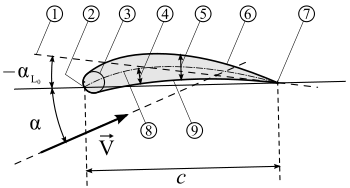Profile geometry – 1: Zero lift line; 2: Leading edge; 3: Nose circle; 4: Camber; 5: Max. thickness; 6: Upper surface; 7: Trailing edge; 8: Camber mean-line; 9: Lower surface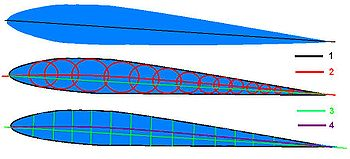Profile lines – 1: Chord, 2: Camber, 3: Length, 4: Midline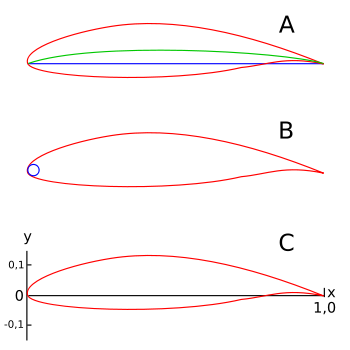A: blue line = chord, green line = camber mean-line, B: leading edge radius, C: x-y-coordinates for the profile geometry (Chord = x-Axis; y-Axis line on that leading edge)

The NACA airfoils are airfoil shapes for aircraft wings developed by the National Advisory Committee for Aeronautics (NACA). The shape of the NACA airfoils is described using a series of digits following the word "NACA." The parameters in the numerical code can be entered into equations to precisely generate the cross-section of the airfoil and calculate its properties.

## Four-digit series

The NACA four-digit wing sections define the profile by:

1. One digit describing maximum camber as percentage of the chord.
2. One digit describing the distance of maximum camber from the airfoil leading edge in tens of percent's of the chord.
3. Two digits describing maximum thickness of the airfoil as percent of the chord.

For example, the NACA 2412 airfoil has a maximum camber of 2% located 40% (0.4 chords) from the leading edge with a maximum thickness of 12% of the chord. Four-digit series airfoils by default have maximum thickness at 30% of the chord (0.3 chords) from the leading edge.

The NACA 0015 airfoil is symmetrical, the 00 indicating that it has no camber. The 15 indicates that the airfoil has a 15% thickness to chord length ratio: it is 15% as thick as it is long.

### Equation for a symmetrical 4-digit NACA airfoil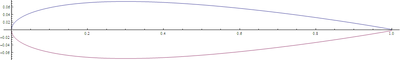Plot of a NACA 0015 foil, generated from formula

The formula for the shape of a NACA 00xx foil, with "xx" being replaced by the percentage of thickness to chord, is:$y_t = \frac{t}{0.2}c\, \left[ 0.2969 \sqrt{\frac{x}{c}} - 0.1260 \left(\frac{x}{c}\right) - 0.3516 \left(\frac{x}{c}\right)^2 + 0.2843 \left(\frac{x}{c}\right)^3 - 0.1015 \left( \frac{x}{c} \right)^4 \right],$

where:

• c is the chord length,
• x is the position along the chord from 0 to c,
• y is the half thickness at a given value of x (centerline to surface), and
• t is the maximum thickness as a fraction of the chord (so 100 t gives the last two digits in the NACA 4-digit denomination).

Note that in this equation, at (x/c) = 1 (the trailing edge of the airfoil), the thickness is not quite zero. If a zero-thickness trailing edge is required, for example for computational work, one of the coefficients should be modified such that they sum to zero. Modifying the last coefficient (i.e. to -0.1036) will result in the smallest change to the overall shape of the airfoil. The leading edge approximates a cylinder with a radius of:$r = 1.1019\; t^2.\,$

Now the coordinates (xU,yU) of the upper airfoil surface, and (xL,yL) of the lower airfoil surface are:$x_U = x_L = x, \qquad y_U = +y_t, \quad \text{and} \quad y_L = -y_t.$

### Equation for a cambered 4-digit NACA airfoil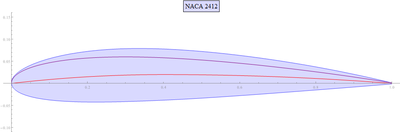Plot of a NACA 2412 foil, including camber line and thickness

The simplest asymmetric foils are the NACA 4 digit series foils, which use the same formula as that used to generate the 00xx symmetric foils, but with the line of mean camber bent. The formula used to calculate the mean camber line is:$y_c = \left\{\begin{array}{ll} \displaystyle{m\, \frac{x }{ p ^2} \left( 2\, p\, - \frac{x}{c} \right)}, & 0 \leq x \leq pc \\ \\ \displaystyle{m\, \frac{c-x}{(1 - p)^2} \left( 1 + \frac{x}{c} - 2\, p \right)}, & pc \leq x \leq c \end{array} \right.$

where:

• m is the maximum camber (100 m is the first of the four digits),
• p is the location of maximum camber (10 p is the second digit in the NACA xxxx description).

For this cambered airfoil, the coordinates (xU,yU) and (xL,yL), of respectively the upper and lower airfoil surface, become:\begin{align} x_U &= x - y_t\, \sin \theta, \qquad & y_U &= y_c + y_t\, \cos \theta, \\ x_L &= x + y_t\, \sin \theta, & y_L &= y_c - y_t\, \cos \theta, \end{align}

where\begin{align} \theta = \arctan{ \left( \frac{dy_c}{dx} \right)} \end{align}

## Five-digit series

The NACA five-digit series describes more complex airfoil shapes:

1. The first digit, when multiplied by 0.15, gives the designed coefficient of lift (CL).
2. Second and third digits, when divided by 2, give p, the distance of maximum camber from the leading edge (as per cent of chord).
3. Fourth and fifth digits give the maximum thickness of the airfoil (as per cent of the chord).

For example, the NACA 12018 airfoil would give an airfoil with maximum thickness of 18% chord, maximum camber located at 10% chord, with a lift coefficient of 0.15

The camber-line is defined in two sections:$y_c = \begin{cases}\frac{k_1}{6}\left\{x^3-3mx^2+m^2(3-m)x\right\}, & 0

where the chordwise location x and the ordinate y have been normalized by the chord. The constant m is chosen so that the maximum camber occurs at x = p; for example, for the 230 camber-line, p = 0.3 / 2 = 0.15 and m = 0.2025. Finally, constant k1 is determined to give the desired lift coefficient; for camber-line 230 again, k1 = 15.957 is used.

## Modifications

Four- and five-digit series airfoils can be modified with a two-digit code preceded by a hyphen in the following sequence:

1. One digit describing the roundness of the leading edge with 0 being sharp, 6 being the same as the original airfoil, and larger values indicating a more rounded leading edge.
2. One digit describing the distance of maximum thickness from the leading edge in tens of percent of the chord.

For example, the NACA 1234-05 is a NACA 1234 airfoil with a sharp leading edge and maximum thickness 50% of the chord (0.5 chords) from the leading edge.

In addition, for a more precise description of the airfoil all numbers can be presented as decimals.

## 1-series

A new approach to airfoil design pioneered in the 1930s in which the airfoil shape was mathematically derived from the desired lift characteristics. Prior to this, airfoil shapes were first created and then had their characteristics measured in a wind tunnel. The 1-series airfoils are described by five digits in the following sequence:

1. The number "1" indicating the series
2. One digit describing the distance of the minimum pressure area in tens of percent of chord.
3. A hyphen.
4. One digit describing the lift coefficient in tenths.
5. Two digits describing the maximum thickness in percent of chord.

For example, the NACA 16-123 airfoil has minimum pressure 60% of the chord back with a lift coefficient of 0.1 and maximum thickness of 23% of the chord.

## 6-series

An improvement over 1-series airfoils with emphasis on maximizing laminar flow. The airfoil is described using six digits in the following sequence:

1. The number "6" indicating the series.
2. One digit describing the distance of the minimum pressure area in tens of percent of chord.
3. The subscript digit gives the range of lift coefficient in tenths above and below the design lift coefficient in which favorable pressure gradients exist on both surfaces
4. A hyphen.
5. One digit describing the design lift coefficient in tenths.
6. Two digits describing the maximum thickness in tens of percent of chord.

For example, the NACA 612-315 a=0.5 has the area of minimum pressure 10% of the chord back, maintains low drag 0.2 above and below the lift coefficient of 0.3, has a maximum thickness of 15% of the chord, and maintains laminar flow over 50% of the chord.

## 7-series

Further advancement in maximizing laminar flow achieved by separately identifying the low pressure zones on upper and lower surfaces of the airfoil. The airfoil is described by seven digits in the following sequence:

1. The number "7" indicating the series.
2. One digit describing the distance of the minimum pressure area on the upper surface in tens of percent of chord.
3. One digit describing the distance of the minimum pressure area on the lower surface in tens of percent of chord.
4. One letter referring to a standard profile from the earlier NACA series.
5. One digit describing the lift coefficient in tenths.
6. Two digits describing the maximum thickness in tens of percent of chord.
7. "a=" followed by a decimal number describing the fraction of chord over which laminar flow is maintained. a=1 is the default if no value is given.

For example, the NACA 712A315 has the area of minimum pressure 10% of the chord back on the upper surface and 20% of the chord back on the lower surface, uses the standard "A" profile, has a lift coefficient of 0.3, and has a maximum thickness of 15% of the chord.

## 8-series

Supercritical airfoils designed to independently maximize airflow above and below the wing. The numbering is identical to the 7-series airfoils except that the sequence begins with an "8" to identify the series.

Wikimedia Foundation. 2010.

### Look at other dictionaries:

• NACA (disambiguation) — NACA may refer to: Contents 1 Organizations 2 Science, technology, and medicine Organizations National Advisory Commit …   Wikipedia

• NACA (National Advisory Committee for Aeronautics) airfoil — A method of indicating characteristics of an airfoil. This can be done by describing the airfoil using 4, 5, 6, 7, or 8 digits. In a NACA 4 digit airfoil, the first digit expresses the camber in the percentage of chord, the second digit gives the …   Aviation dictionary

• Airfoil — An airfoil (in American English) or aerofoil (in British English) is the shape of a wing or blade (of a propeller, rotor or turbine) or sail as seen in cross section. An airfoil shaped body moved through a fluid produces a force perpendicular to… …   Wikipedia

• NACA-Profil — Geometrie eines Profils – 1: Nullauftriebslinie (zero lift line); 2: Flügelnase (leading edge); 3: Krümmungsradius der Flügelnase (nose circle); 4: Skelettlinie (camber); 5: maximale Profildicke (max. thickness); 6: obere Profilseite (upper… …   Deutsch Wikipedia

• NACA-Profile — Geometrie eines Profils – 1: Nullauftriebslinie (zero lift line); 2: Flügelnase (leading edge); 3: Krümmungsradius der Flügelnase (nose circle); 4: Wölbung (camber); 5: maximale Profildicke (max. thickness); 6: obere Profilseite (upper surface);… …   Deutsch Wikipedia

• NACA cowling — Curtiss AT 5A Hawk with NACA cowling at the Langley Memorial Aeronautical Laboratory, October 1928 …   Wikipedia

• NACA cowling — A type of cowling most widely used on reciprocating radial engines. It is a drag reducing annular cowling whose trailing edge fairs smoothly into the fuselage or the engine nacelle. The airfoil shape of a cowling produces a forward dynamic force… …   Aviation dictionary

• Supercritical airfoil — it has since been mainly applied to increase the fuel efficiency of many high subsonic aircraft. Research in 1940 by DVL s K. A. Kawalki led to subsonic profiles very similar to the supercritical profiles, which was the basis for the objection in …   Wikipedia

• National Advisory Committee for Aeronautics — NACA redirects here. For other uses, see NACA (disambiguation). National Advisory Committee for Aeronautics NACA The official seal of NACA, depicting the Wright brothers first flight at Kitty Hawk …   Wikipedia

• Eastman Jacobs — Eastman N. Jacobs (1902 ndash;1987) was a leading aerodynamicist who worked for NACA s Langley Research Center from the 1920s to the 1940s. He was responsible for advancing many fields in aerodynamics, dealing particularly with wind tunnels,… …   Wikipedia